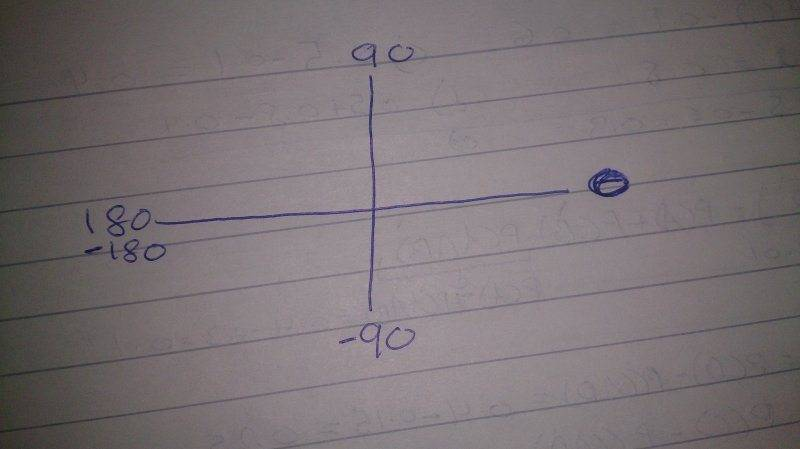# Simple trigonometry question

Member warned about not using the homework template
In trigonometry equation (finding theta):
1) If the range of theta includes negative and positive part (such as -360<=theta<=360), and I got a value of 90,180,270 or 360.. Do I have to write the answer twice positive and negative?

2) if range of theta is positive only, and I got a negative value of theta, do I have to do 360 + (negative value of theta)? .. To make the answer positive

BvU
Homework Helper
1) no, all ##\theta## are in range (but see comment (*))
2. yes, repeatedly if necessary (you may find ##\theta## = -3000) -- the wording here is confusing: -120 would have to be reported as -120 + 360 = 240.

(*)
Make a drawing of the unit circle. ##-360 \le \theta\le +360## seems unlogical. Could it be ##-180 < \theta \le 180 ## ?

1) no, all ##\theta## are in range (but see comment (*))

(*)
Make a drawing of the unit circle. ##-360 \le \theta\le +360## seems unlogical. Could it be ##-180 < \theta \le 180 ## ?
But suppose the range is -180<=theta<=180
If i got a value of 90 or 180, would it be + and negative?

For the -360<=theta<=360 it's like two ranges : one is from 0 to 360, the other from 0 to -360, so two drawings.. Or maybe I have to write -360<=2theta<=360

BvU
Homework Helper
But suppose the range is -180<=theta<=180
If i got a value of 90 or 180, would it be + and negative?
The 90 is positive and 90 - 360 is out of range. So +90 only.
The 180 is on the bound. Your bounds coincide, so both -180 and +180 are in range. But there is only one answer, so the overlapping bounds are not a good idea. That's why I used ##-180 < \theta \le 180##.

The 90 is positive and 90 - 360 is out of range. So +90 only.
The 180 is on the bound. Your bounds coincide, so both -180 and +180 are in range. But there is only one answer, so the overlapping bounds are not a good idea. That's why I used ##-180 < \theta \le 180##.
But consider this graph, isn't it possible to write -90?BvU